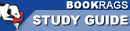Study Guide

# Journey Through Genius: The Great Theorems of Mathematics Setting & Symbolism

This Study Guide consists of approximately 26 pages of chapter summaries, quotes, character analysis, themes, and more - everything you need to sharpen your knowledge of Journey Through Genius.
 This section contains 183 words (approx. 1 page at 400 words per page) View a FREE sample## Pythagorean Theorem

The theorem that expresses the proportions of the sides of a right triangle. The theorem is that the square of the hypotenuse is equal to the sum of the squares of the two perpendicular legs and is written a² = b² + c² .

The procedure of determining the area of a closed polygon by constructing a square of the same area.

## Prime Number

A number that can only be divided evenly by itself and 1.

## Binomial Theorem

A theorem refined by Isaac Newton that allows for the expansion of algebraic equations with exponents.

## Harmonic Series

The series 1 + 1/2 + 1/3 + 1/4 + ... The sum of the series diverges toward infinity

## π

The Greek letter "pi." π is the ratio between the diameter and the circumference of a circle.

## Transfinite Cardinal

A number used to describe types of infinite sets.

## Cubic Equation

A function of the form ax³ + bx² + cx + d where a is not equal...

 This section contains 183 words (approx. 1 page at 400 words per page) View a FREE sample